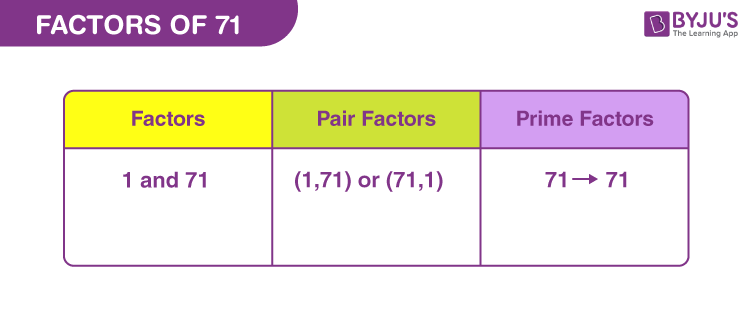# Factors of 71

Factors of 71 are the natural numbers that divide the original number, evenly. Thus, these factors are also called the even divisors of the original number. Since 71 is a prime number, therefore, it is divisible by 1 and the number itself.

We can also say, if the product of two natural numbers is equal to 71, then the two numbers are the factors of 71. Let us learn here how to find these factors.## How to Find the Factors of 71?

71 is a prime number, therefore it will be divisible by 1 and itself. Hence, there will be no remainder left after division.

 71 ÷ 1 = 71 [Remainder = 0] 71 ÷ 71 = 1 [Remainder = 0]

Therefore, there are only two factors of 71. They are 1 and 71.

Let us see if 71 is divisible by any other number.

71 ÷ 2 = 35.5

71 ÷ 5 = 14.2

71 ÷ 11 = 5.9167

71 ÷ 22 = 3.227

Thus, we can see, we are not able to find any other factors here.

## Pair Factors of 71

Pair factors are the numbers that on multiplication results in the original number.

• 1 × 71 = 71
• 71 × 1 = 71

Therefore, the pair factors of 71 are (1, 71) or (71, 1).

If we consider the negative factors in pairs to get 71, then;

• -1 × -71 = 71
• -71 × -1 = 71

Therefore, the negative pair factors are (-1, -71) or (-71, -1).

## Prime Factorisation of 71

The prime factor of 71 is 71 only, because it cannot be divided by any other prime number.

71 ÷ 71 = 1

 Prime factorisation of 71 = 71 Exponential form = 711

## Solved Examples

Q.1: What is the sum of all the factors of 71?

Solution: The factors of 71 are 1 and 71.

Sum = 1 + 71 = 72

Therefore, the required sum is 72.

Q.2: What are the common factors of 71 and 73?

Solution: We have to list the factors of 71 and 73.

71 → 1, 71

73 → 1, 73

Therefore, the common factor of 71 and 73 is 1.

Q.3: What are the greatest common factors of 71 and 72?

Solution: The factors of respective numbers are:

71 → 1, 71

72 → 1, 2, 3, 4, 6, 8, 9, 12, 18, 24, 36, 72

Therefore, GCF of 71 and 72 is 1.

### Practice Questions

1. What are the divisors of 71?
2. Find the GCF of 71 and 51.
3. What are the common factors of 71 and 91?
4. What is the average of factors of 71?
5. What are the pair factors of 71?

## Frequently Asked Questions on Factors of 71

### What are the factors of 71?

The factors of 71 are 1 and 71.

### Is 71 a prime number?

Yes, 71 is a prime number because it has only two factors.

### Is 71 divisible by any other factor?

71 is only divisible by 1 and 71, but not by any other number.

### What are the multiples of 71?

The first ten multiples of 71 are 71, 142, 213, 284, 355, 426, 497, 568, 639 and 710.

### What is the prime factorisation of 71?

The prime factorisation of 71 is 71.